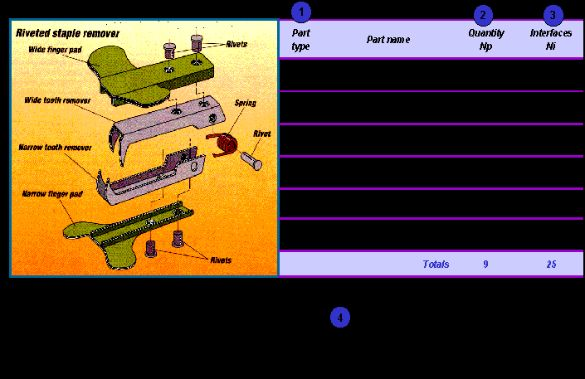## Boothroyd & Dewhurst complexity factor

Provides a numerical indication of the relative complexity of an assembly. The number alone is of little value, unless used to support comparison with alternative solutions. Thus, the complexity factor could be used as part of the analysis of competitive products or to evaluate alternative design concepts.

### Number of part types = Nt

Each new part can be considered as a new part type - 5 M8x10 screws = 1 part type

### Number of parts = Np

The total number of components in the assembly - 5 M8x10 screws = 5 parts

### Number of interfaces = Ni

It helps to consider each part in turn - how many other parts have an interface with it? This approach is simple, but can result in some interfaces being counted twice, so care should be taken to ensure that the same approach is applied consistently

### Calculate the complexity factor

Using the equation shown in the figure.### Notes

• Need a calculator
• Best done as a team exercise - engineering & production
• Can focus on the number rather than the understanding gained about the assembly through calculating it
• Need to apply consistently for comparisons